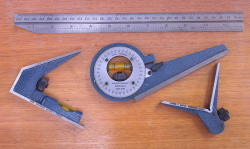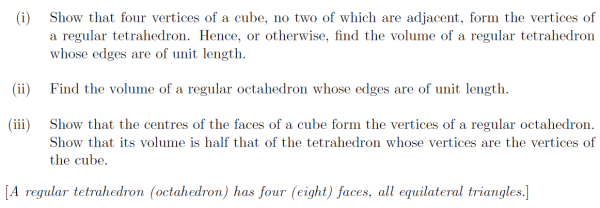# Geometry and Advanced Mathematics Exams Questions

##### Age 16 to 18
Many students are a bit put off by geometry problems, because they can sometimes seem unfamiliar and you might be unsure about what exactly you are being asked to do. We hope that by reading this article and working through some of the ideas within it, you will feel more confident about tackling geometry questions.First and foremost, it is almost always useful to draw at least one clear large diagram when solving a geometry problem. It is rare that a STEP or other advanced mathematics examinations question will include a diagram; it is more likely to consist of a description of a mathematical situation that could be represented diagrammatically. In A Guide to Problem Solving, we suggest that the first steps to solving any mathematics problem is to understand it, and devise a plan. For geometry questions, a diagram helps tremendously with the understanding - seeing that two lines are parallel, or being able to observe where a circle's tangents might be, can often be much more useful than just knowing it from words on a page. It's best to make your diagram quite big so that it doesn't become cluttered. It can also be useful to have your diagram on a separate sheet from your working, so that you can refer to it easily.Another important exercise when solving a geometry problem is to make sense of what you know. Which areas of geometry might be useful? Are you working with coordinates? Are there circles? Which lines are parallel? Could you represent the situation using vectors? The problem may well involve geometry ideas that you learned when you were quite a bit younger. Here's something to try: get some sticky notes and write down all the useful geometry facts you can think of, one fact on each note. Then arrange them on a desk together with related facts. Hold this image in your mind when tackling a geometry challenge, so that you have all the relevant facts close to hand. Then take a look at our Useful Things to Remember to see if you came up with the same facts we did!

Let's take a look together at an example from STEP Paper III, 1998:To start with, think about what you know, and what mathematics you might need to use. Once you've had a chance to think, look below to see my thoughts:

I have a cube, so I can use facts about right angles. There are probably going to be right-angled triangles, so I might need to use Pythagoras. It's worth taking the time to draw a diagram, but since we're in three dimensions, it might be easier to interpret if I come up with a coordinate system to refer to each relevant point. Then I might be able to use vectors or coordinate geometry. Finally, there might be pyramids involved, so I recall that the volume of a pyramid is one third of its base area multiplied by the perpendicular height.

Now see if you can plan out what you might do for each stage of the question. Once again, after you've thought about it, click below to see my plan:

Part (i)
A regular tetrahedron has four equilateral triangular faces, so if I can show that all the edges are the same I'll know the triangles are equilateral and I'm done. Then I think I can work out the volume by subtracting some volumes from the cube, these will be nice right-angled pyramids and thus easy to work out, and then what's left is the regular tetrahedron.

Part (ii)
Split the octahedron into two square-based pyramids. Pythagoras's theorem will allow me to work out all of the relevant lengths.

Part (iii)
Work out the vertices of the octahedron by finding the coordinates of the midpoints of each face. Then show that the appropriate lengths are equal again, so we have equilateral triangles. Finally, use the volumes calculated in part (i) and (ii) with appropriate scaling, to get the required result.

Once you've had a go at the question for yourself, you can take a look at this solution. Then why not have a go at some other STEP questions involving Geometry